# Sigma Notation Terminology

Previous: Introduction to Sigma Notation

Next: Sigma Notation Properties

## Terminology

The infinite sumcan be written in a more compact way. Using the capital greek letter sigma,, we may write this sum asWe often use the following terminology when using this notation

• the general term of the sum is• the index of summation is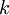• the limits of summation are 1 and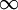, although in general the limits can be any integer,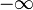, or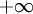.

## Simple Example

Consider the infinite seriesIn this example, the general term is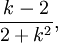the index of summation is, and the limits of summation are 3 and infinity.

Previous: Introduction to Sigma Notation

Next: Sigma Notation Properties